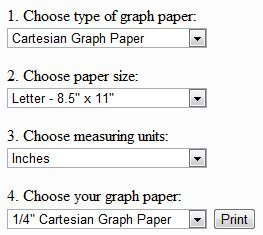Editor Ratings:
User Ratings:
[Total: 0 Average: 0]

Here are 10 free websites to print graph paper. These websites let you get free graph paper by just printing the graph paper that you want. Almost all types of free graph paper can be created by using these websites.

## 1. Print Free Graph Paper:

This is quite a simplistic graph paper print website with a sole purpose of creating printable graph paper. It supports many different types of graph papers. You can choose the graph paper type, graph paper size, and grid size, and this website will let you print that graph paper.Here are the various graph paper types available for print:

• Cartesian Graph Paper
• Engineering graph paper
• Polar graph paper
• Isometric graph paper
• Logarithmic graph paper
• Hexagonal graph paper
• Probability graph paper
• Smith Chart graph paper

## 2. Incompetech Free Online Graph Paper:

This website has the most exhaustive collection of printable graph papers that I have come across. You can print graph paper of almost any type with this free graph paper website. You start by selecting your graph paper type, and then customize the graph paper as per your needs. You can choose paper size of your graphing paper, specify border, choose line thickness of free graph paper, and even specify line color. It will then generate graph paper in PDF format that you can download, and then print free graph paper.

Here are the free graph paper types supported by this website:

Square Graph Papers:

• Grid
• Multi-weight Grid
• Dots
• Crosses
• Light Verticals
• Grid Lined
• Axonometric Perspective

Triangle and Hexagonal Graph Paper:

• Equilateral Triangle
• Octagonal
• Hexagonal
• Iso-Dots
• Tumbling Block
• Diamond – Trapezoid
• Hex Dot
• Semi-bisected Trapezoid
• Equilateral Triangle Dots
• Variable Triangle

Writing and Note-taking:

• Notebook
• Lined
• Double Lined Penmanship
• Cornell Lined
• Cornell Graph
• 3-Line Penmanship
• Cornell Music
• Chinese Character Guides
• Calligraphy Guideline
• Genkoyoushi

Circular and Polar Graph Paper:

• Circular – Grid Pattern
• Polar Graph Paper
• Circular – Hex Pattern

Brick, Asymmetic, and Specialty Graph Paper:

• Asymmetric
• Brick
• Moorish
• Accounting – Ledger
• Log / Semi-Log
• Polar
• Storyboard
• “174 Paper”
• Engineer’s Paper
• Music Notation
• Fretboard Diagrams
• Number Line
• Celtic Knot
• Perspective

Try this free graph paper printer here.

Also check out free organizer for students.

## 3. Printable Graph Paper

This is another website that provides free graphing paper. Unlike the websites mentioned above, it does not offers any customization in the graph papers that can be printed. It provides many pre-configured variations of graph paper in PDF format. You just need to download the appropriate PDF graph paper that you want, and then print graph paper. It primarily provides rectangular and square graph papers, alongwith some speciality graph papers. Specialty graph papers include Impedance graph paper, Smith Chart, Compact circles, overlapping circles, Triangle, Trigonometry degrees and radians, and Probability Graph Paper. Try it free here.

## 4. Graph Paper Generator:

This is another free online graph paper generator, but comes with very limited options. You can generate only Square graph paper with this, and can have only 4, 5, or 6 squares per inch. It does offers option to specify color of grid lines for your free graphing paper. Try free here.

## 5. Mathbits Printable Graph Paper:

Mathbits provides a nice collection of graph papers in PDF format. It does not offers any customization, but it has a nice collection of specialty graph papers. Many graph papers include labeled scales. It also includes graphs with scatter template plots, T1-Nspire clickpad, Trigonometric graph papers, Polar graph papers, Dot papers, and papers with number lines. Try free here.

## 6. Vertex Excel based Graph Paper template:

Vertex42 provides excel based graph paper template. It includes square, triangular, and isometric graph paper templates. Download free here.

## 7. Teaching Tools Graph Paper:

Teaching Tools also provides a free graph paper generator to print graph paper. It provides only square graph paper, but you can do lots of customization on the graph paper. You can specify number of rows, number of columns, row height, column width, line thickness, and line color. Try free here.

## 8. PrintARuler Graph paper:

PrintARuler has started building a collection of printable graphing papers in PDF format. Currently, it provides square graph papers and logarithmic graph papers. All graph papers are available as PDF file. Download graph paper free here.

Update (1-Sep-2012): This website is in process of re-branding, and no graph papers are available at the moment.

## 9. Intmath Graph Paper:

Intmat provides a very limited number of printable PDF graph papers. It includes rectangular graph paper, Semi-Logarithmic graph paper, Log-Log graph paper, and Polar graph paper. Download here.

## 10. Create Your Own Graph Paper in Excel:

If you use Microsoft Excel, or some Excel alternative, you can easily create your own graph paper. Here are two articles that explain how to use excel to create your own printable graphing paper: 1, 2.

All these free graph paper resources help you print graph paper for free, and create almost any type of graph paper. Some other educational resources include 3D programming language for kids, and free online lectures.

 Editor Ratings: User Ratings:[Total: 0 Average: 0] Free/Paid: Free Tags: graph paperstudent